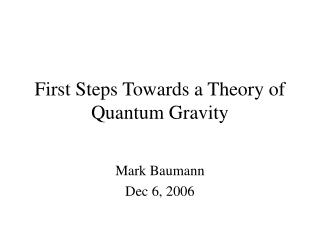DownloadDownload PresentationFirst Steps Towards a Theory of Quantum Gravity

# First Steps Towards a Theory of Quantum Gravity

Download Presentation## First Steps Towards a Theory of Quantum Gravity

- - - - - - - - - - - - - - - - - - - - - - - - - - - E N D - - - - - - - - - - - - - - - - - - - - - - - - - - -
##### Presentation Transcript

1. First Steps Towards a Theory of Quantum Gravity Mark Baumann Dec 6, 2006

2. Where to Begin? How might we quantize gravity? Can we describe gravitational interaction by exchange of a massless particle? If so, what kind of particle? Guess #1: Scalar (spin-0) particle “Einstein-Fokker theory” Problem: No gravitational bending of light or precession of Mercury’s orbit Guess #2: Vector particle, spin-1 Problem: Gravity exhibits repulsion

3. Where to Begin? Guess #3: Vector particle, spin-2 The “graviton” Perturbative approach: graviton is a perturbation to a flat background spacetime, like a gravitational wave Another approach: Start with GR and modify it to make it look more like quantum field theory.

4. General Relativity in a Nutshell Einstein’s Field Equations: “Matter tells space how to curve, and curved space tells matter how to move.” --J.A. Wheeler RHS: Matter/energy LHS: Curvature of space Curvature of space is encapsulated in the “metric” g, which tells us how far apart things are. The metric is found by solving Einstein’s field equations.

5. General Relativity in a Nutshell Metric in particle physics: “Minkowski” metric that describes Cartesian space and includes special relativity Metric in GR: We said we were going to modify GR. How?

6. Spin and Torsion • Fundamental properties of a particle include: mass, spin • GR couples mass and the metric, a geometric property of spacetime • Right now, GR is solely a macroscopic theory. However, for a quantum theory of gravity, incorporating spin is essential! • How could we couple spin to the geometry of spacetime? • Idea: Couple spin to torsion • To understand torsion, we start with the covariant derivative and the connection

7. Covariant Derivatives Covariant Derivative in quantum field theory: “Converts” global gauge invariance into local gauge invariance. Covariant Derivative in general relativity: connection The connection allows you to compare vectors from different tangent spaces. The covariant derivative is defined so that it’s a tensor. We give these the same name because they are, in fact, the same thing! The GR version is more general.

8. Mathematical Roadmap Riemannian Manifold Physics done here Distance & Curvature Add a metric Manifold with Connection Covariant Derivatives Add a connection Manifold More abstraction More structure Make it locally “flat” Topological Space Add a topology Limits & Continuity Set

9. Torsion Defined i.e. - it is a measure of the non-commutativity of the lower two indices of the connection. Torsion is a tensor quantity, unlike the connection. Geometrically, the torsion measures how much rotation a vector undergoes when you parallel transport it from one tangent space to another. Idea (due to Cartan, 1922): Couple spin and torsion

10. Connection In classical GR, we solve the Einstein equations for the metric. The standard choice for a connection in standard GR is the Levi-Civita connection, sometimes called “Christoffel symbols.” We get this connection if we start with a metric and assume the connection is torsion-free (and also “metric-compatible”). Now we are supposing the connection might not be torsion-free. By allowing the possibility of torsion, we are “freeing” the connection to be another variable. Whereas before we solved the Einstein equations for the metric, now we wish to solve a new set of equations for both the metric and the connection. But which equations?

11. Einstein-Cartan Equations Einstein’s equations are derived by varying the Einstein-Hilbert action: where V is a 4-d spacetime volume and the Lagrangian = R, which is the Ricci curvature scalar, which depends on the connection.  If the connection depends on torsion, then so does R. This gives us the Einstein-Cartan action:

12. Einstein-Cartan Equations Varying the Einstein-Cartan action via the usual process, we get the Einstein-Cartan equations: The first equation has reproduced Einstein’s equations. The second equation involves a spin density tensor S and a modified torsion tensor T, and therefore couples spin with torsion.

13. Coupling Spinors to GR Quantum field theory uses a flat metric, but we don’t know how to do QFT on a curved spacetime. So, why not make the metric flat? In GR, we can make the metric look flat at any point by a change of basis. In other words: This new basis e is called the tetrad or vielbein basis, first introduced by Weyl (1929). Now we can proceed normally! However, what are the consequences of this change of basis?

14. Tetrad Formulation At every spacetime point, we have a different basis. One consequence: the Dirac matrices are no longer constant, but depend on position as follows:  Every occurrence of  should be preceded by e Once we find the Dirac matrices, we can compute the metric through the usual equation:  We solve for the metric by solving for the  ‘s

15. Further Reading Baez, J. and Muniain, J. Gauge Fields, Knots, and Gravity, volume 4 of Series on Knots and Everything. World Scientific, 1994. -- Written by a mathematician, mathematically rigorous but intuitively presented. Culminates with Ashtekar’s New Variables. Carroll, Sean M. Spacetime and Geometry: An Introduction to General Relativity. Addison Wesley, 2003. -- A mathematical introduction to GR. Good descriptions, includes tetrad formulation. Ortín, Tomás. Gravity and Strings. Cambridge University Press, 2004.